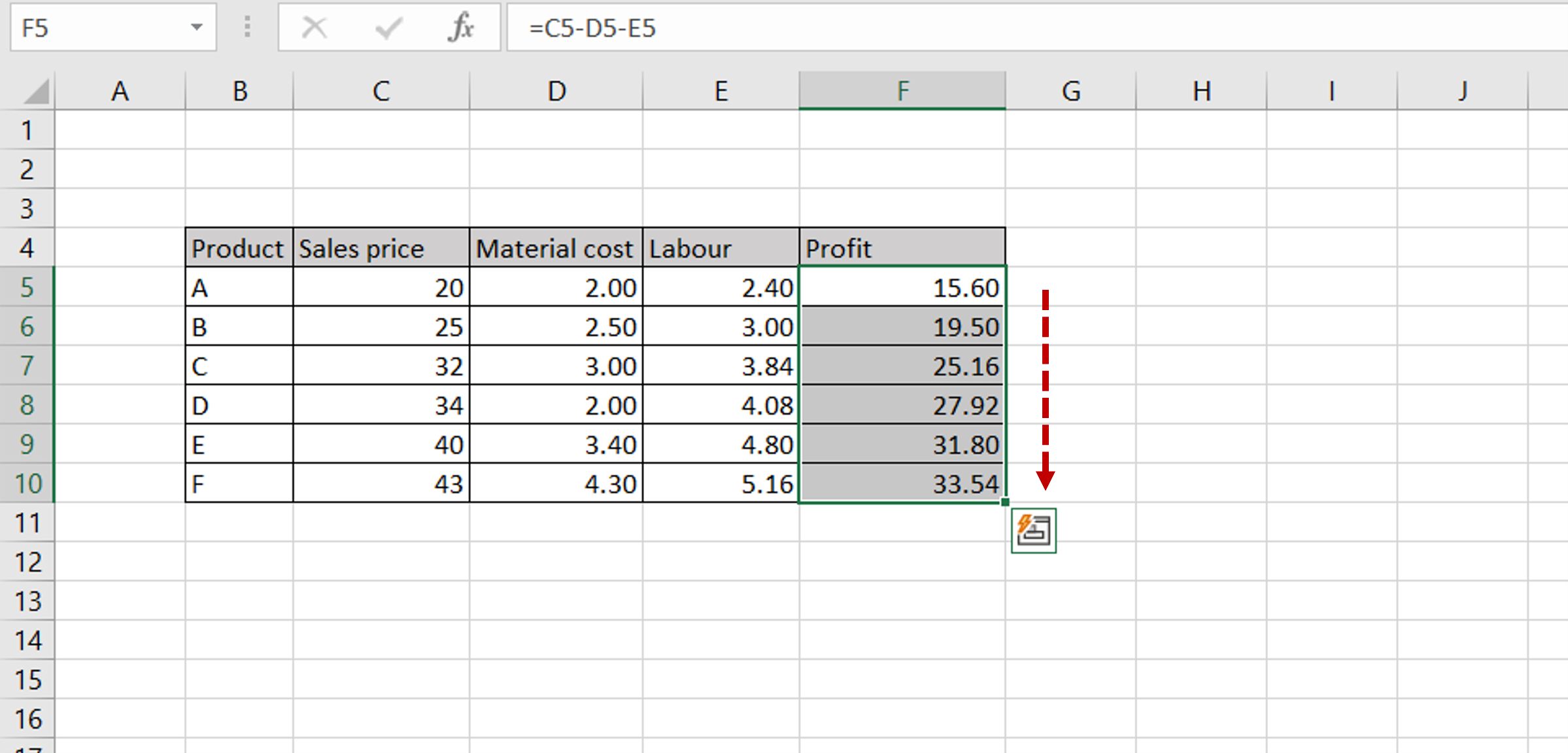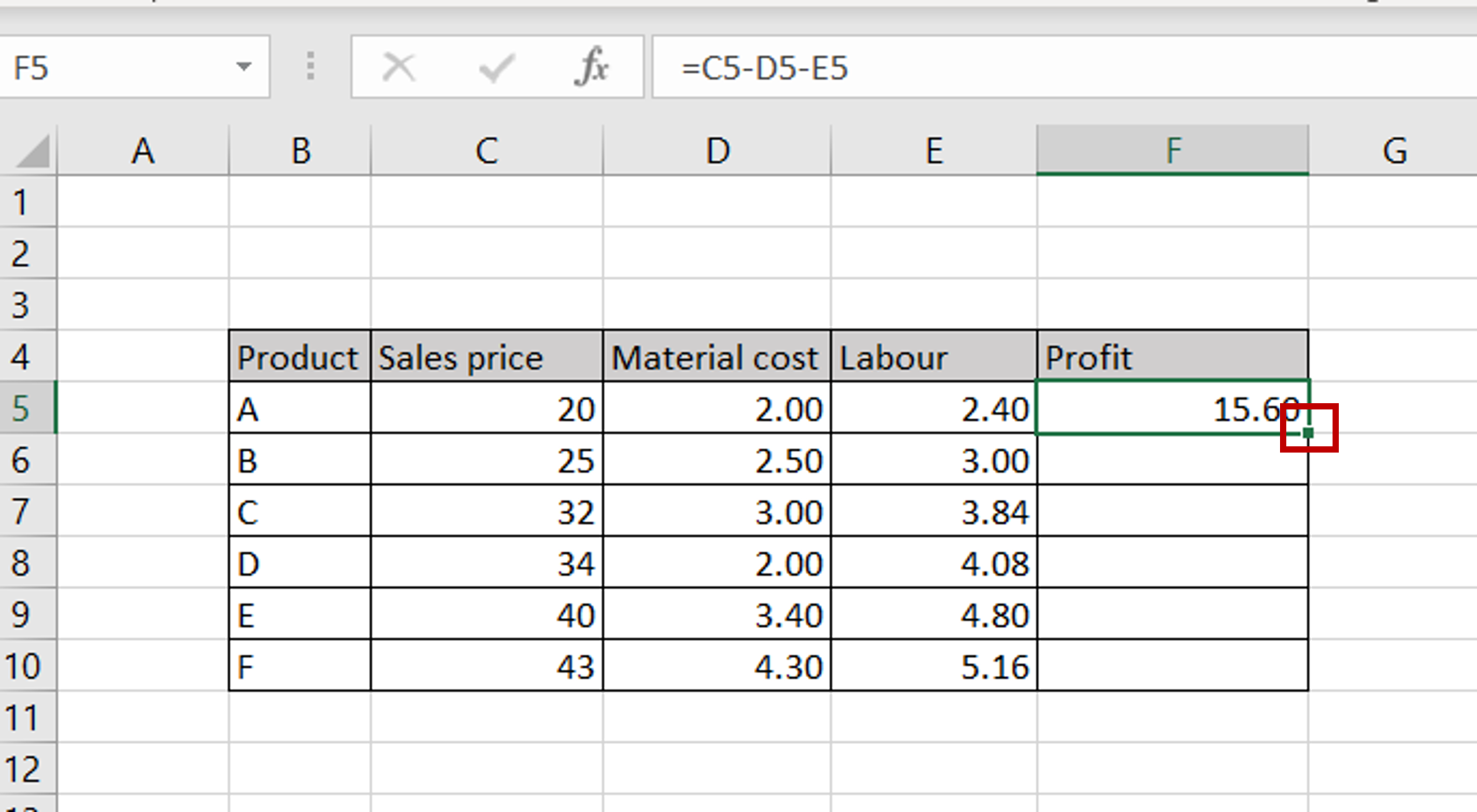# How to drag a formula in Excel

You can watch a video tutorial here.Excel is widely used when creating tables in which columns have to be calculated. Not only can a formula be created for the calculation, but the calculation can be copied to other cells. You may have a table in which the Sales and Costs are given and the Profit has to be derived from these numbers. The advantage of using Excel is that a formula can be created in the first cell of the column and then dragged to the remaining cells of the column.

### Step 1 – Create the formula– Type the formula in the first cell of the column of computed values

### Step 2 – Select the formula– Select the cell that has the formula
– Position the cursor on the small box in the lower right corner of the cell (this is known as the fill handle) and it will change to a plus sign (+)

### Step 3 – Drag the formula– Click and drag the fill handle down to the end of the column
– The formula will be copied to the other cells in the column